## Combining Predicates

On this page, you will combine predicates using Boolean functions to write on the stage in three colors.

### Boolean Functions

At the very lowest level, computer circuitry is made of wires, and each wire is either on or off. So the only operations that can be performed at that lowest level are those that operate on single-bit values (just ones and zeros, that is, just ons and offs). These are called logical (or Boolean) functions. (They're predicates, because their range is Booleans, but these are special in that their domain is also Booleans.) There are three Boolean functions in the Operators palette: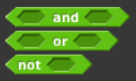AAP-2.F.5, AAP-2.F.1
Notice that both the blocks themselves and the input slots in the blocks are hexagonal. Boolean functions take Boolean values (True or False) as inputs and report a new Boolean value as output. When you use Boolean functions in a program, though, the inputs will usually be expressions such as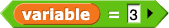.

1. Experiment with different input values in all three blocks, and take notes about the results you get.

Instead of dragging a true or false block into the input slot of an and, or, or not block, you can click the empty input slot to control a true/false toggle: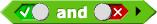The and and not blocks work exactly the way you'd expect from the meanings of those words in English:

AAP-2.F.3
•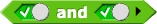reports true, and any other combination of inputs reports false.
• AAP-2.F.2
•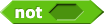reports the opposite of whichever input value you use.
AAP-2.F.4
But or is a little different in computer science. In English, the word "or" has two different meanings:
• Either but not both: such as "Let's check whether it will be rainy or sunny tomorrow." In computer science, this is called exclusive or because one option excludes the other.
• Either or both: such as "Ask your teacher if you have any questions or if you need help." This is called inclusive or because it's possible for both options to be included.

1. Experiment. Does the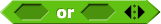block implement exclusive or inclusive or?
AAP-2.F.1
The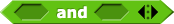,, andblocks will appear as
AND
,
OR
, and
NOT
and will work exactly the same way as they do in Snap!.
1. Make the blocks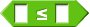,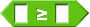, and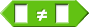. (You can copy the characters ≤, ≥, and ≠ from this page and paste them into the Snap! Make-a-block window.)
2. Build a predicate that tells whether an input is between two other inputs, and test it with several different cases.
You can decide whether between? will include the two boundary inputs or not.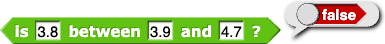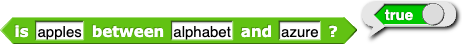#### Making a Predicate

• Choose the hexagonal predicate shape.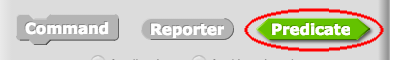• You must use the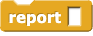block to report the result of reporters (including predicates).
3. Use between? to create a script that lets you write on the stage in three colors (depending on the height on the stage), using your mouse.
4. AAP-2.E part b
There's no built in ≤ block in Snap!. Suppose we wanted to build one. Which two of the following Boolean expressions are equivalent to the expression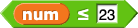?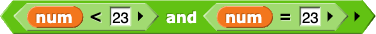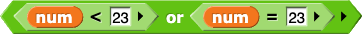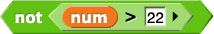5. AAP-2.F part b
Which of the following expressions will report true?
1.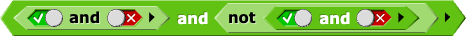2.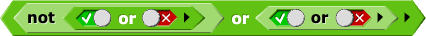I only
II only
I and II
Neither I nor II
Brian, OK, I went a little over the top here, but some teachers might like to dig in too. Please review this table carefully; it would be easy to get something wrong. --MF, 1/14/20
TG (for answer key): i) The and function can be represented as TFFF; ii-v) Answers will vary for parts ii and iii. The following table lists the 16 (part iv) possible two-input Boolean operators and their usefulness. The goal is for students to think about usefulness—not for them to accurately categorize each of the functions or even to learn the logic of each one. Unless you have a background in logic or computer science, even you (as teacher with the answer key) are likely to find some of these take a bit of thinking to wrap your mind around.
Useful? Why?
TTTT no always reports true
TTTF yes equivalent to or
TTFT yes equivalent to converse implication (false only when the first input is false but the second input is true; the second input being true implies the first input must be true)
TTFF no reports the value of the first input regardless of the second input, so you could just use the first input as the predicate instead of building this predicate function
TFTT yes equivalent to implication (false only when the first input is true but the second input is false; the first input being true implies the second input must be true)
TFTF no reports the value of the second input regardless of the first input, so you could just use the second input as the predicate instead of building this predicate function
TFFT yes checks whether two inputs are the same (equivalent to = and to XNOR, exclusive NOT OR)
TFFF yes equivalent to and
FTTT yes equivalent to NAND (that is, not (and))
FTTF yes checks whether two inputs are the different (equivalent to not(=) and to XOR, exclusive OR)
FTFT no reports the value of the opposite of the second input regardless of the first input, so you could just use the opposite of the second input as the predicate instead of building this predicate function
FTFF yes equivalent to nonimplication (true only when the first input is true but the second input is false; the first input being true implies the second input must be false)
FFTT no reports the value of the opposite of the first input regardless of the second input, so you could just use the opposite of the first input as the predicate instead of building this predicate function
FFTF yes equivalent to converse nonimplication (true only when the first input is false but the second input is true; the second input being true implies the first input must be false)
FFFT yes equivalent to NOR (that is, not(or))
FFFF no always reports false
vi) There are 256 three-input Boolean operators because there are eight combinations of inputs for a three-input Boolean operator and each of those combinations could report one of two values (T or F): 2^8=256. (For comparison, there are 16 two-input Boolean operators because there are four combinations of inputs for a two-input operator and each can report one of two values (T or F): 2^4=16.)
1. Because there are only two Boolean values (true and false), there are only four combinations of inputs for a two-input Boolean operator. Here are the four combinations shown with the or operator: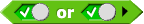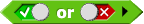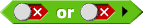1. With or, the four combinations report true, true, true, and false in that order. (Check for yourself that this is true.) So, you can represent the or function as TTTF (using T and F as abbreviations for true and false). What are the four letters for the and function? (Assume the same ordering of the inputs: TT, TF, FT, and FF as shown above with or.)
2. Make up another set of four letters (such as TFTF), and build a predicate function with that behavior. Describe how the function behaves.
Click to see an example showing and describing the behavior of TFTF.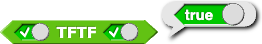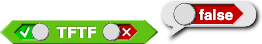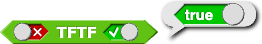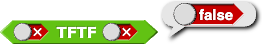The TFTF function reports true whenever the second input is true no matter what the first input is. It's not a useful function because you could just use the second input as the predicate instead of building this predicate function.
3. Do the same thing with another set of four letters.
4. How many possible two-input Boolean operators are there?
5.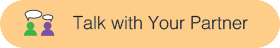Obviously, TTTF (the or function) is useful, but TTTT is not useful (why not?). Which of the possible two-input Boolean operators do you think would be useful? Discuss why.
6. How many three-input Boolean operators are there?